DiscretePlotComp.mw

 > restart;

 > with( plots ):

 > with( plottools ):

 >

Shrinking Sphere Problem

Comparison between

Plotting a Discrete Sampling of Points

and Plotting a Geometric Object

9 February 2007

 >

The point P and the curves Q and R

 > P := r -> [ 0, 0, r ];

 >

 > Qr := (r,a) -> r*sqrt(4*a^2-r^2)/2/a:

 > Qx := Qr(r,a)*cos(theta):

 > Qy := r^2/2/a:

 > Qz := Qr(r,a)*sin(theta):

 > Q := unapply( [ Qx, Qy, Qz ], [theta,r,a] );

 >

 > Rr := (r,a) -> sqrt( 4*a^2-r^2 ):

 > Rx := r*sqrt(4*a^2-r^2)*cos(theta)/(2*a-sqrt(4*a^2-r^2)*sin(theta)):

 > Ry := r^2                         /(2*a-sqrt(4*a^2-r^2)*sin(theta)):

 > Rz := 0:

 > R := unapply( [ Rx, Ry, Rz ], [theta,r,a] );

 >

Plotting routines

Animations

 > anim1  := (r,a,n) -> animate( plotPQR, [ theta, r, a ], theta=0..2*Pi, frames=n, background=P1(r,a,n),  args[4..-1] ):

 > anim1p := (r,a,n) -> animate( plotPQR, [ theta, r, a ], theta=0..2*Pi, frames=n, background=P1p(r,a,n), args[4..-1] ):

 >

 > anim1p( 1, 1, 21, scaling=constrained ); # circles Q and R plotted as discrete samples of points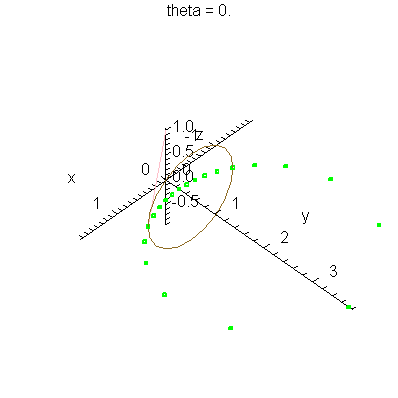> anim1( 1, 1, 21, scaling=constrained );  # circles Q and R plotted as circles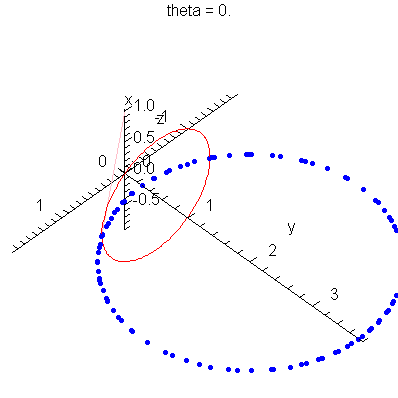>

 > anim1p( 1, 2, 21, scaling=constrained ); # circles Q and R plotted as discrete samples of points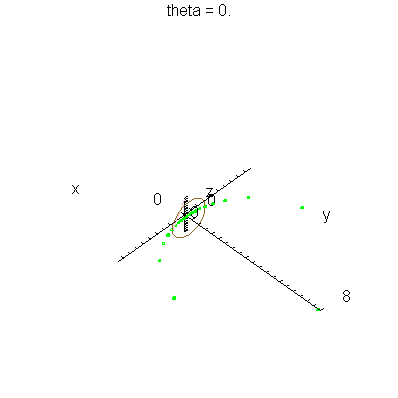> anim1( 1, 2, 21, scaling=constrained );  # circles Q and R plotted as circles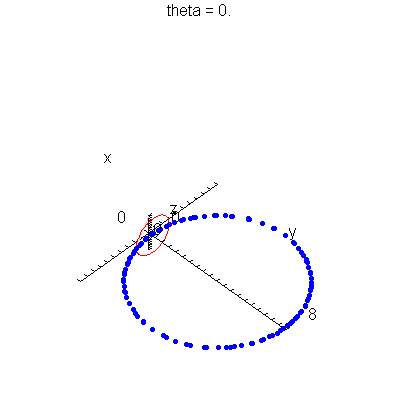>

 > anim1p( 1, 2, 41, scaling=constrained ); # circles Q and R plotted as discrete samples of points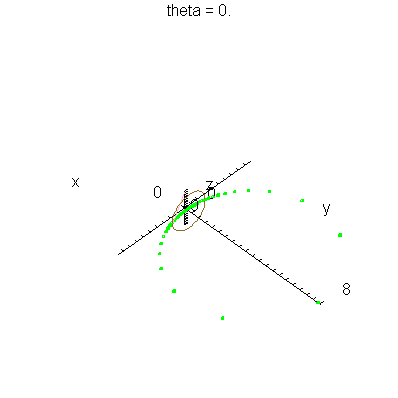> anim1( 1, 2, 41, scaling=constrained );  # circles Q and R plotted as circles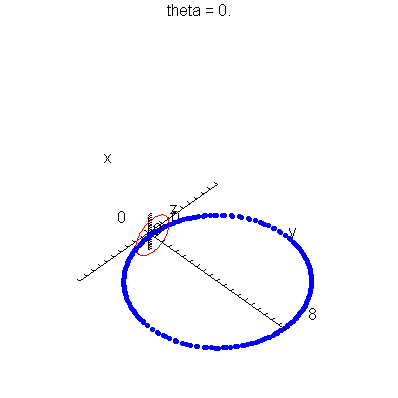>

 >

 >